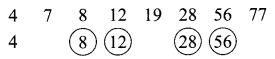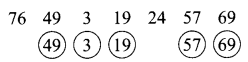# DAV Class 5 Maths Chapter 3 Worksheet 3 Solutions

The DAV Class 5 Maths Book Solutions Pdf and DAV Class 5 Maths Chapter 3 Worksheet 3 Solutions of Multiples and Factors offer comprehensive answers to textbook questions.

## DAV Class 5 Maths Ch 3 Worksheet 3 Solutions

Question 1.
Encircle the even numbers.
4  7  8  12  19  28  56  77
Solution:Question 2.
Encircle the odd numbers.
76  49  3  19  24  57  69
Solution:Question 3.
Fill in the blanks.
(a) 75 is an _______ number (even/odd).
Solution:
odd

(b) 178 is an _______ number (even/odd).
Solution:
even

(c) 1,082 is an ______ number (even/odd).
Solution:
even

(d) 1,493 is an ______ number (even/odd).
Solution:
odd

(e) A number that is a multiple of ______ is called an even number.
Solution:
2

(f) Smallest even number: _____
Solution:
2

(g) Smallest odd number: ______
Solution:
1DAV Class 5 Maths Chapter 3 Worksheet 3 Notes

• Even numbers: Even numbers are those numbers that are multiples of 2.
e.g., 2, 4, 6, 8, 10, …… are even numbers.
• Odd numbers: Odd numbers are those numbers that are not multiple of 2.
e.g., 1, 3, 5, 7, 9, …… are odd numbers.